# 2.6: A Particle in a Multidimensional Box

•• Valeria D. Kleiman
• University of Florida
$$\newcommand{\vecs}{\overset { \rightharpoonup} {\mathbf{#1}} }$$ $$\newcommand{\vecd}{\overset{-\!-\!\rightharpoonup}{\vphantom{a}\smash {#1}}}$$$$\newcommand{\id}{\mathrm{id}}$$ $$\newcommand{\Span}{\mathrm{span}}$$ $$\newcommand{\kernel}{\mathrm{null}\,}$$ $$\newcommand{\range}{\mathrm{range}\,}$$ $$\newcommand{\RealPart}{\mathrm{Re}}$$ $$\newcommand{\ImaginaryPart}{\mathrm{Im}}$$ $$\newcommand{\Argument}{\mathrm{Arg}}$$ $$\newcommand{\norm}{\| #1 \|}$$ $$\newcommand{\inner}{\langle #1, #2 \rangle}$$ $$\newcommand{\Span}{\mathrm{span}}$$ $$\newcommand{\id}{\mathrm{id}}$$ $$\newcommand{\Span}{\mathrm{span}}$$ $$\newcommand{\kernel}{\mathrm{null}\,}$$ $$\newcommand{\range}{\mathrm{range}\,}$$ $$\newcommand{\RealPart}{\mathrm{Re}}$$ $$\newcommand{\ImaginaryPart}{\mathrm{Im}}$$ $$\newcommand{\Argument}{\mathrm{Arg}}$$ $$\newcommand{\norm}{\| #1 \|}$$ $$\newcommand{\inner}{\langle #1, #2 \rangle}$$ $$\newcommand{\Span}{\mathrm{span}}$$$$\newcommand{\AA}{\unicode[.8,0]{x212B}}$$

In quantum mechanics it is easy to expand the number of dimensions where a particle can exist.

## 2-Dimensional box

Consider a particle that can move in 2 independent directions, $$x,y$$. When each coordinate can be treated independently, the solution to the 2-D Schcrödinger equation yields a wavefunction $$\psi(x,y)$$ which depends on both coordinates and its characterized by 2 independent quantum numbers $$n_x$$ and $$n_y$$.

$\Psi_{n_x,n_y}(x,y)= \psi_{n_x}(x) \cdot \psi_{n_y}(y) = \sqrt{\dfrac{2}{L_x}\cdot \dfrac{2}{L_y} } \cdot \sin(\dfrac{n_x\pi}{L_x}x) \cdot \sin(\dfrac{n_y\pi}{L_y}y)$

The energy of the states depends on those two quantum numbers

$E_{n_x,n_y} = \dfrac{h^2}{8mL^2_x}n^2_x+\dfrac{h^2}{8mL^2_y}n^2_y= \dfrac{h^2}{8m}\cdot (\dfrac{n^2_x}{L^2_x}+\dfrac{n^2_y}{L^2_y})$

where $$m$$ is the mass of the particle, $$L_x$$ and $$L_y$$ are the length of the box in the $$x$$ and $$y$$ directions, respectively. Each state of the system is given by a specific combination of quantum numbers $$n_x$$ and $$n_y$$ which are integer numbers that vary independently.

Looking at a square box (where $$L_x =L_y =L$$ we can evaluate the probability density and the energy of each state, with

$|\Psi_{n_x,n_y}(x,y)|^2 = |\dfrac{2}{L} \cdot \sin(\dfrac{n_x\pi}{L}x) \cdot \sin(\dfrac{n_y\pi}{L}y)|^2 \hspace{2cm} E_{n_x,n_y}=\dfrac{h^2}{8mL^2}\cdot (n^2_x+n^2_y)$.

The presence of two quantum numbers provides the possibility of degeneracy, which occurs when different states, each one with its own probability density, have the same total energy. In this case, the states described by $$\Psi_{a,b}(x,y)$$ and $$\Psi_{b,a}(x,y)$$ will have different probability densities, but the same energy.

 $$n_x,n_y$$ $$\Psi_{n_x,n_y}(x,y)|^2$$ $$E_{n_x,n_y}$$ (1,1)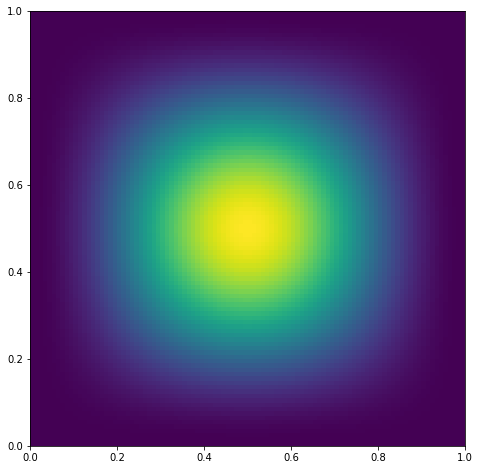$$E_{1,1}= 0.752\ eV$$ (1,2)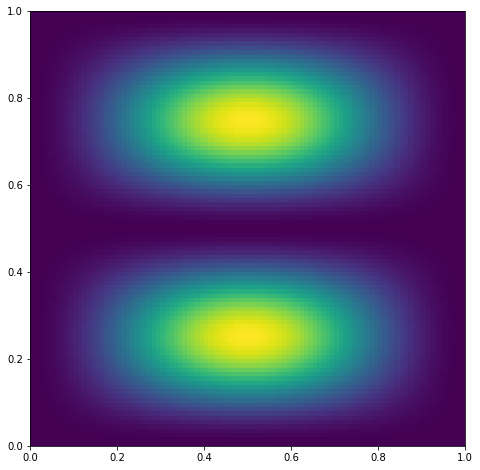$$\textcolor{darkblue}{E_{1,2}= 1.880\ eV}$$ (2,1)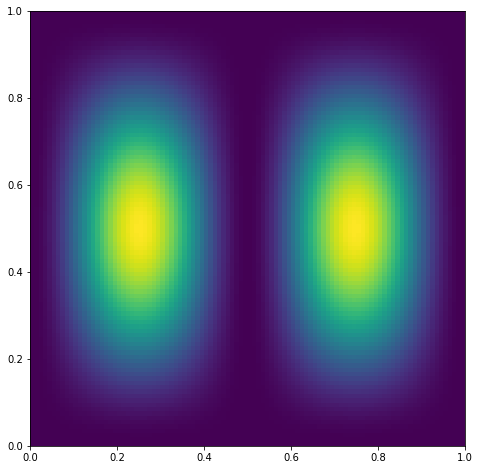$$\textcolor{darkblue}{E_{2,1}= 1.880\ eV}$$ (2,2)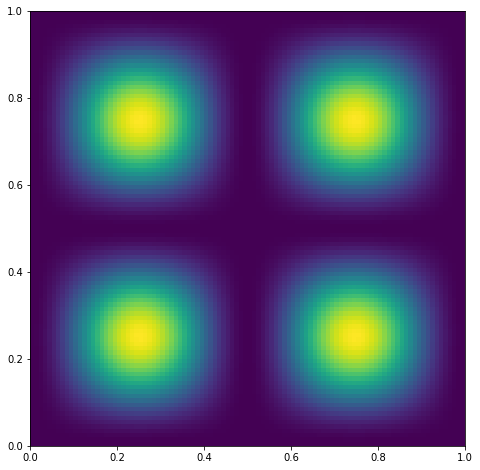$$E_{2,2}= 3.008\ eV$$ (1,3)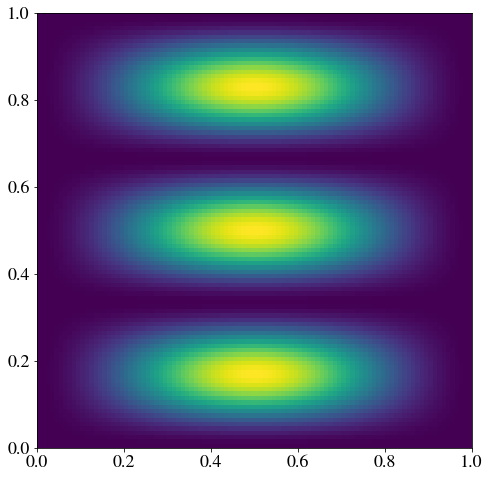$$\textcolor{darkgreen}{E_{1,3}= 3.761\ eV}$$ (3,1)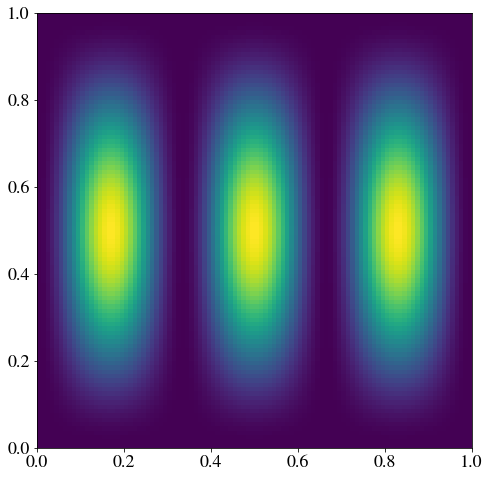$$\textcolor{darkgreen}{E_{3,1}= 3.761\ eV}$$ (2,3)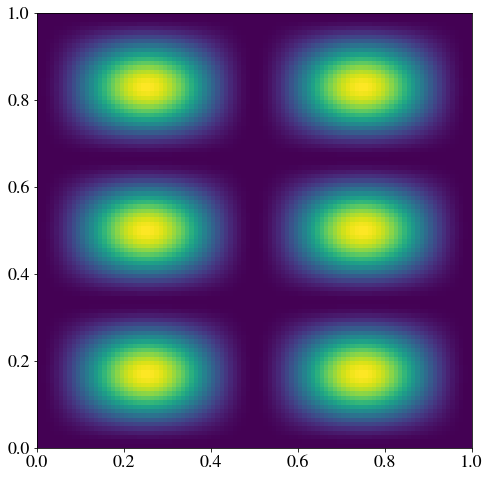$$\textcolor{darkmagenta}{E_{2,3}= 4.889\ eV}$$ (3,2)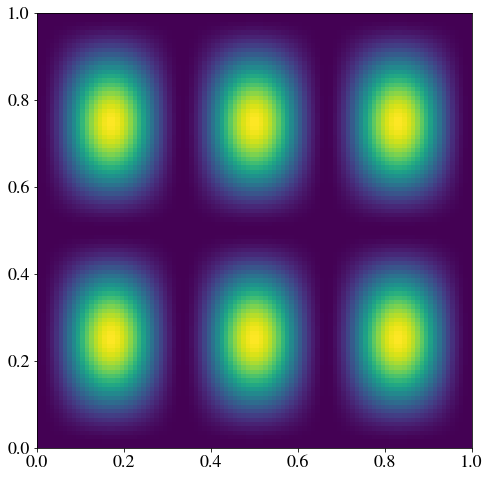$$\textcolor{darkmagenta}{E_{2,3}= 4.889\ eV}$$

## 3-Dimensional box

Moving into 3 dimensions is done in a similar fashion, where the wavefucntion will depend on 3 coordinates ($$x,y,z$$); three independent quantum numbers will identify each state ($$n_x,n_y,n_z)$$ and the energies will dependend on those 3 independent quantum numbers (each one with values $$1, 2, ...,\infty$$. The additional dimension increases the possibility of more degenerate states. For example, for a cubic box (all side lenghts being equal), we obtain $$E_{1,3,6}= E_{1,6,3}=E_{3,6,1}=E_{3,1,6}=E_{6,1,3}=E_{6,3,1}$$, although each of those states will have a different probability density in space.

This page titled 2.6: A Particle in a Multidimensional Box is shared under a not declared license and was authored, remixed, and/or curated by Valeria D. Kleiman.